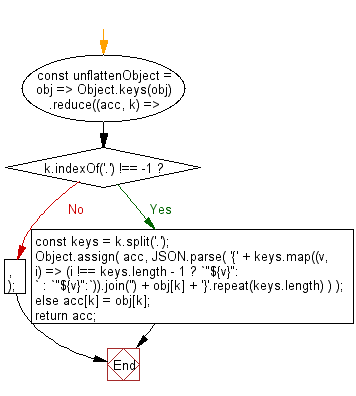# JavaScript: Unflatten an object with the paths for keys

## JavaScript fundamental (ES6 Syntax): Exercise-112 with Solution

Write a JavaScript program to unflatten an object with the paths for keys.

• Use nested Array.prototype.reduce() to convert the flat path to a leaf node.
• Use String.prototype.split('.') to split each key with a dot delimiter and Array.prototype.reduce() to add objects against the keys.
• If the current accumulator already contains a value against a particular key, return its value as the next accumulator.
• Otherwise, add the appropriate key-value pair to the accumulator object and return the value as the accumulator.

Sample Solution:

JavaScript Code:

``````//#Source https://bit.ly/2neWfJ2
const unflattenObject = obj =>
Object.keys(obj).reduce((acc, k) => {
if (k.indexOf('.') !== -1) {
const keys = k.split('.');
Object.assign(
acc,
JSON.parse(
'{' +
keys.map((v, i) => (i !== keys.length - 1 ? `"\${v}":{` : `"\${v}":`)).join('') +
obj[k] +
'}'.repeat(keys.length)
)
);
} else acc[k] = obj[k];
return acc;
}, {});
console.log(unflattenObject({ 'a.b.c': 1, d: 1 }));
```
```

Sample Output:

```{"a":{"b":{"c":1}},"d":1}
```

Flowchart:Live Demo:

See the Pen javascript-basic-exercise-112-1 by w3resource (@w3resource) on CodePen.

Improve this sample solution and post your code through Disqus

What is the difficulty level of this exercise?

Test your Programming skills with w3resource's quiz.

﻿

## JavaScript: Tips of the Day

How to remove a specific item from an array?

Find the index of the array element you want to remove using indexOf, and then remove that index with splice.

The splice() method changes the contents of an array by removing existing elements and/or adding new elements.

```const array = [2, 5, 9];

console.log(array);

const index = array.indexOf(5);
if (index > -1) {
array.splice(index, 1);
}

// array = [2, 9]
console.log(array);```

The second parameter of splice is the number of elements to remove. Note that splice modifies the array in place and returns a new array containing the elements that have been removed.

For the reason of completeness, here are functions. The first function removes only a single occurrence (i.e. removing the first match of 5 from [2,5,9,1,5,8,5]), while the second function removes all occurrences:

```function removeItemOnce(arr, value) {
var index = arr.indexOf(value);
if (index > -1) {
arr.splice(index, 1);
}
return arr;
}

function removeItemAll(arr, value) {
var i = 0;
while (i < arr.length) {
if (arr[i] === value) {
arr.splice(i, 1);
} else {
++i;
}
}
return arr;
}
//Usage
console.log(removeItemOnce([2,5,9,1,5,8,5], 5))
console.log(removeItemAll([2,5,9,1,5,8,5], 5))
```

Ref: https://bit.ly/2N9nKRp